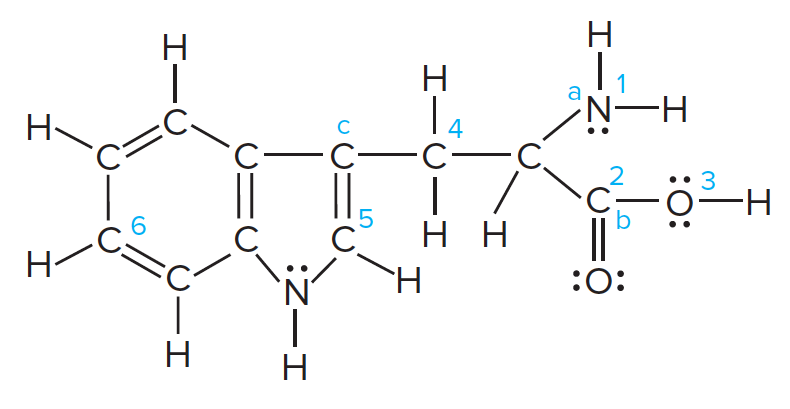# Problem: Tryptophan is one of the amino acids found in proteins:Predict the bond angles at points a, b, and c.

###### FREE Expert Solution
90% (316 ratings)
###### Problem Details

Tryptophan is one of the amino acids found in proteins:Predict the bond angles at points a, b, and c.

Frequently Asked Questions

What scientific concept do you need to know in order to solve this problem?

Our tutors have indicated that to solve this problem you will need to apply the Bond Angles concept. You can view video lessons to learn Bond Angles. Or if you need more Bond Angles practice, you can also practice Bond Angles practice problems.

What textbook is this problem found in?

Our data indicates that this problem or a close variation was asked in Chemistry: The Molecular Nature of Matter and Change - Silberberg 8th Edition. You can also practice Chemistry: The Molecular Nature of Matter and Change - Silberberg 8th Edition practice problems.